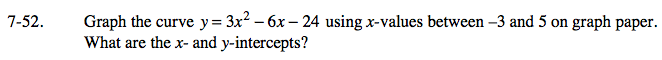Home > CAAC > Chapter 7 > Lesson 7.1.5 > Problem7-52

7-52.

Graph the curve y = 3x2 − 6x − 24 using x-values between −3 and 5 on graph paper. What are the x- and y-intercepts? Homework Help ✎Use a table to find points on the graph by substituting values between −3 and 5 into
the equation, then graph the curve and find the intercepts.

Use the eTool below to help you solve this problem.
Click the link at right for the full version of the eTool: AC 7-52 HW eTool